# Structures

A structure is really just a compound data type. It’s a way of grouping several related variables under a common variable name. For example, if you were writing a program that involved employee data, you would have several individual pieces of data to store regarding a given employee. You would have a last name, first name, salary, and so on. If you place all that data into separate variables, it may become difficult to keep track of everything as your program grows in complexity. Which variables in your program are relevant to employee data and which are related to something else? This will become an even worse problem when you consider that other parts of your program might need similar data. For example, you would also need to store customer data, which would also have fields such as last name. Which last name goes with employees and which with customers? A structure provides you a fairly easy way to group all the data together. The following example illustrates a generic definition of a structure, and a specific one.

Generic example:

`struct structname {   vartype varone;   vartype vartwo; };`

Specific example:

`struct employee {  char lastname;  char firstname ;  float salary; };`

Once you have defined a structure you can then declare a variable of that structure type anywhere you wish. You create an instance of this data type just like you would create an instance of one of C++’s standard data types. You have the data type name followed by some name you wish to give your variable. Just like all variables, you have allocated a space in memory set aside to hold data of a certain type. The space you allocated depends on the size of your structure. You determine the size of a structure by totaling all of the individual data types. For example, if

your structure contains two ints and one char, then it will be 2 * (four bytes per int + 1 byte for the character = 9 bytes).

`employee myemployee;`

You can then access the elements of the structure by using the name of the structure variable you created followed by a period and then the name of the element of the structure you wish to access.

`myemployee.salary = 40000.44`

As you can see, the structure definition is rather simple. The keyword struct is followed with the name of the structure. That name can be any valid C++ name, just as you would name any other variable. You then have brackets that define the boundaries of the structure. Because structures are often used in several places in a program, they are often defined in a header file. You can then simply include that header file in any source file where you wish to use the structure. The following example uses a structure to store data.

### Example 8.1

Step 1: Type the following code into your favorite text editor.

`#include <fstream> #include <stdexcept> #include <iostream> using namespace std;   struct division {  float dividend;  float divisor;  float answer; }; int main ()  {  division localdivide;  try {    cout << "Please enter a number. \n";    cin >> localdivide.dividend;    cout << "Please enter another number.\n";    cin >> localdivide.divisor;        localdivide.answer =   localdivide.dividend/localdivide.divisor;        cout << localdivide.dividend << "divided by " ;    cout << localdivide.divisor << " is " <<    localdivide.answer;    }// end of the try blcok         catch(…) {   cout << "an error occurred!"; } return 0;    }`

Step 2: Compile the code.

Step 3: Run the code. You should see something similar to Figure 8.1.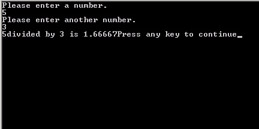Figure 8.1: Using a structure.

As you can see, structures are not more difficult than standard C++ data types. However, a structure is a powerful tool because it allows you to group logically related data under a single name.

What is even more interesting is that a structure can also be passed into a function. This is of particular use when you have several variables that need to be passed into a function. If you consider our previous examples, you should recall a few that had several functions that required several items to be passed to them. Passing more than three or four variables to a function can be convoluted and confusing. It becomes an exceedingly inelegant-looking program. However, you can pass a single variable, a structure that contains all the various pieces of data you require. Passing them in a structure can be very convenient.

As was previously stated, you can use a structure just like any other data type. This means that not only can it be passed to a function as an argument, but also it can be returned. This can be very useful. Recall that functions can only return a single item. What if your function needs to give back several pieces of data? Returning a structure will allow that to happen. The following example illustrates this concept.

### Example 8.2

Step 1: Place the following code in your favorite text editor.

`#include <cmath> #include <iostream> using namespace std;   // define the structures struct geometry_data {  float radius;  double angle; }; struct geometry_answers {  float area;  double sine;  double cosine;  double tangent; }; // prototype functions geometry_answers compute(struct geometry_data mystruct); int main ()  {  geometry_data input;  geometry_answers output;  cout << "Enter the radius of the circle \n";  cin >> input.radius;  cout << "Enter the angle in rads \n"; cin >> input.angle;  output = compute(input);  cout << " The area is "<< output.area << "\n";      cout << " The sine of the angle is " << output.sine <<  "\n";        cout << " The cosine of the angle is " <<  output.cosine << "\n";  cout << " The tangent of the angle is " <<     output.tangent << "\n"; return 0; } geometry_answers compute(struct geometry_data mystruct) {  geometry_answers answer;  answer.area = 3.14f * pow(mystruct.radius,2);  answer.sine = sin(mystruct.angle);  answer.cosine = cos(mystruct.angle);  answer.tangent = tan(mystruct.angle);  return answer; };`

Step 2: Compile the code.

Step 3: Execute the code. You should see something much like what is shown in Figure 8.2.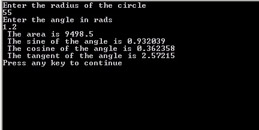Figure 8.2: Structures as return types.

You can see how one structure is used to pass data into the function, then another structure is used to pass out the various answers. In this way, you can do several related geometric operations inside a single function and return all the relevant answers.

You can also pass that structure by reference (recall passing by reference was introduced in the final section of Chapter 3), then it becomes even more convenient. Let’s look at an example.

### Example 8.3

Step 1: Enter the following code in your favorite text editor.

`#include <iostream> using namespace std;   struct triangle {  float base;  float height;  float area; }; void trianglearea(struct triangle &area); int main ()  {  triangle mytriangle;  cout << "Please enter the base of a triangle \n";  cin >> mytriangle.base;  cout << "Please enter the height of a triangle \n";  cin >> mytriangle.height;    trianglearea(mytriangle);  cout << "The area is " << mytriangle.area << " \n";     return 0; } void trianglearea(triangle &area) {  area.area = .5f * (area.base * area.height); }`

Step 2: Compile the code.

Thus, you can see that passing the entire structure by reference accomplishes two goals. First of all, you can pass multiple values in a single variable name. Second, you can get multiple return values stored in a single structure. Without the use of a structure, any function can only return one value. Even with a structure the function can still only return one variable, but now that variable can hold more values.

In an earlier chapter you were introduced to the use of functions such as memcpy in your applications; however, the use of these functions with structures is slightly different. As you have seen, a structure is a complex data type that holds several other simple data types. This has an effect when using some of the built-in functions in C++, such as memcpy. When you use memcpy with an array you simply pass it the destination array, source array, and size of array. When you use memcpy with a structure you must pass it the address of the structure. This is illustrated in the following example.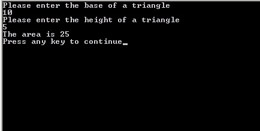Figure 8.3: Passing a structure.

### Example 8.4

Step 1: Enter the following code into a text editor and save it as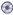08-04.cpp.

`#include <memory> #include <iostream> using namespace std;   struct mystruct { int i;  int x;  int y; };   int main() {  mystruct source,destination;  cout << "Please enter a number \n";  cin >> source.i;  cout << "Please enter another number \n";  cin >> source.x;  cout << "Please enter another number \n";  cin >> source.y;  memcpy(&destination,&source,sizeof(source));  cout << destination.i << endl;  cout << destination.x << endl;  cout << destination.y << endl;  return 0;    }`

Step 2: Compile and run your code. You should see something similar to Figure 8.4.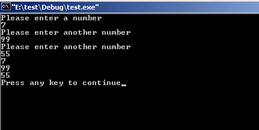Figure 8.4: Structures and memcpy.

You will notice that we copied the contents from the source structure to the destination structure and then the contents of the destination structure are printed. This illustrates that the copy worked.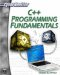C++ Programming Fundamentals (Cyberrookies)
ISBN: 1584502371
EAN: 2147483647
Year: 2005
Pages: 197
Authors: Chuck Easttom